# RD Sharma Solutions for Class 6 Maths Chapter 10: Basic Geometrical Concepts Exercise 10.3

The students can learn about the types of curves and polygons and methods of constructing them in this exercise. RD Sharma Solutions Class 6 has answers explained in a simple language which matches the understanding capacity of students. By understanding the concepts using the solutions PDF, students can analyse their areas of weakness and work on them as per the CBSE marks weightage. Students who aspire to score well in the exam can download RD Sharma Solutions for Class 6 Maths Chapter 10 Basic Geometrical Concepts Exercise 10.3 PDF which are provided here.

## RD Sharma Solutions for Class 6 Maths Chapter 10: Basic Geometrical Concepts Exercise 10.3 Download PDF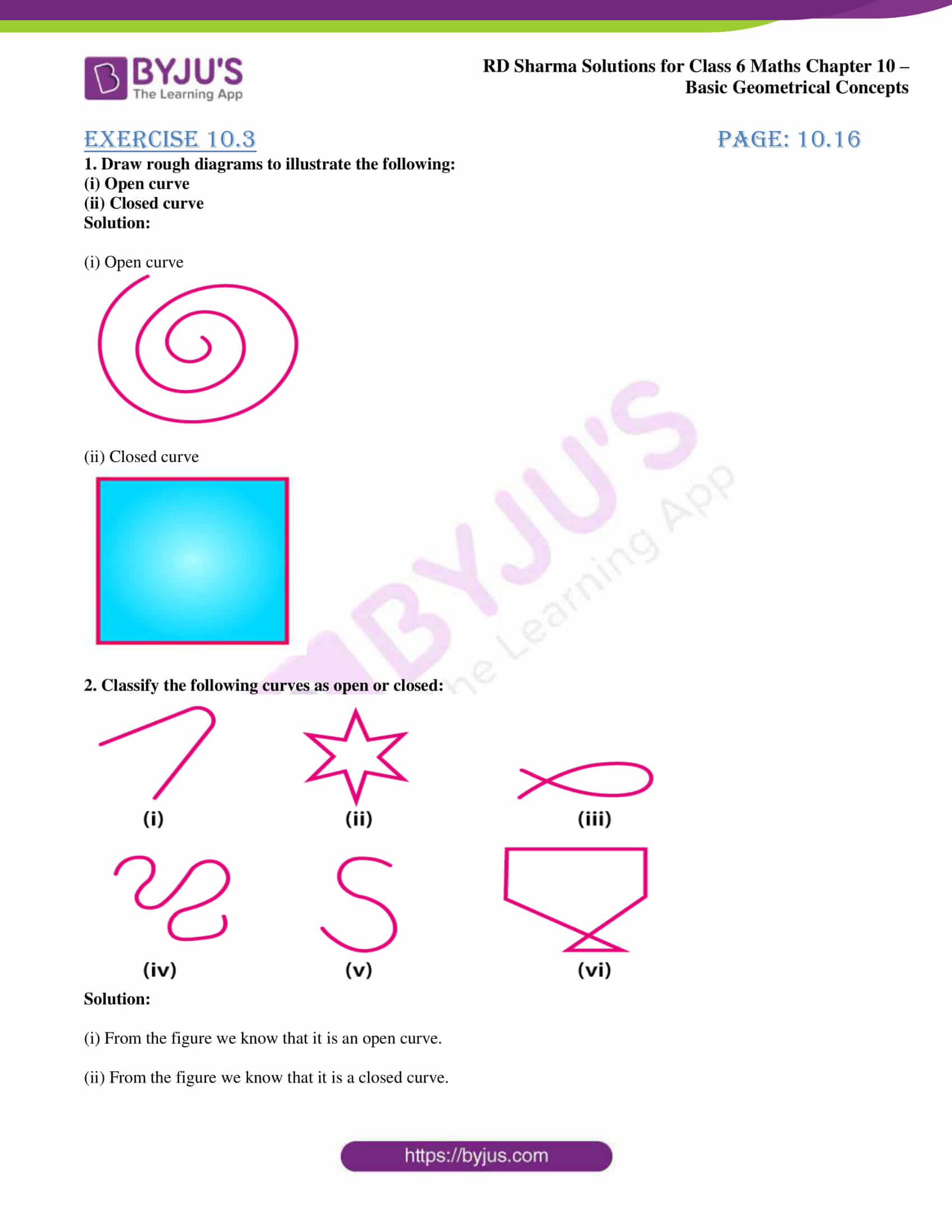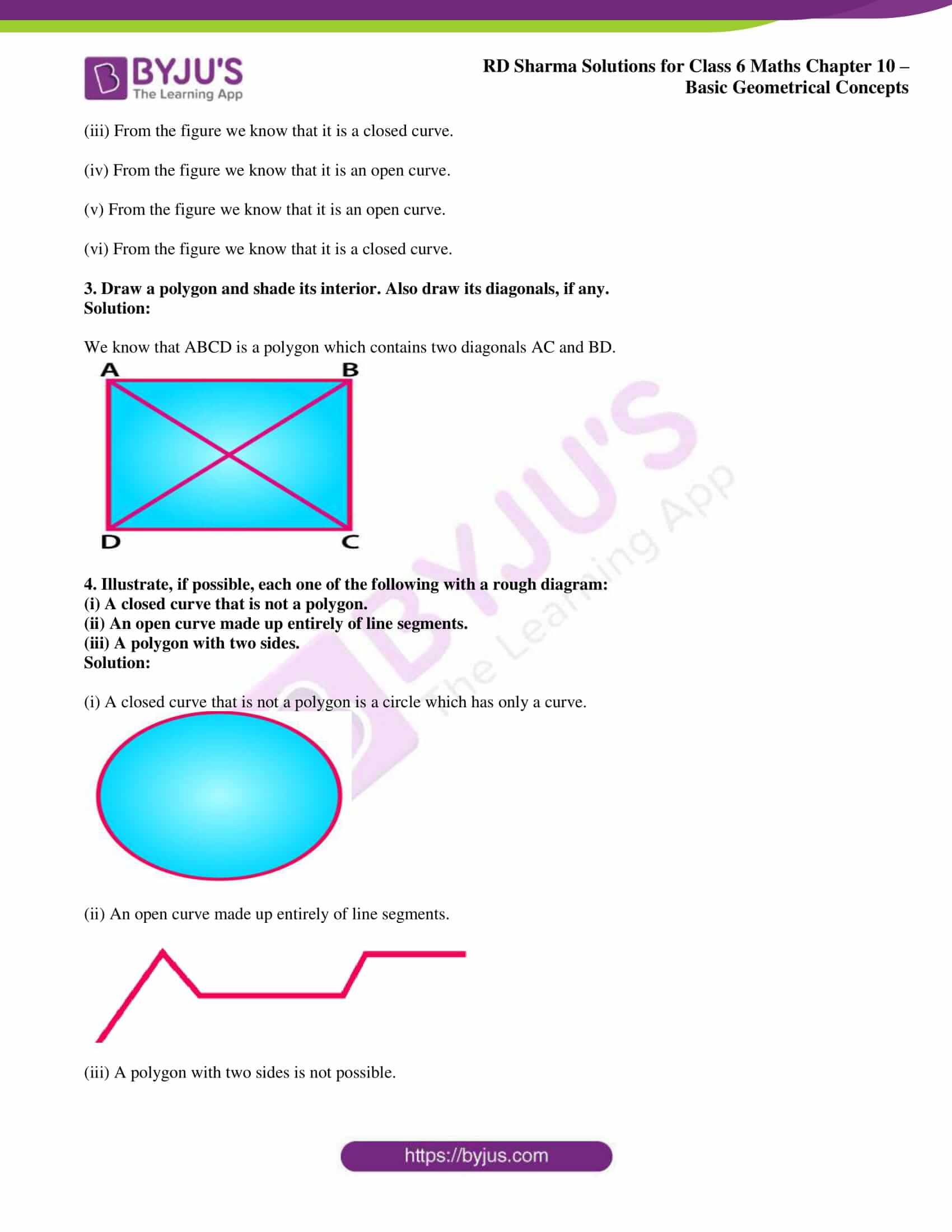### Access answers to Maths RD Sharma Solutions for Class 6 Chapter 10: Basic Geometrical Concepts Exercise 10.3

1. Draw rough diagrams to illustrate the following:

(i) Open curve

(ii) Closed curve

Solution:

(i) Open curve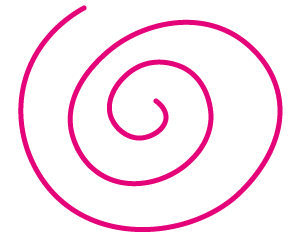(ii) Closed curve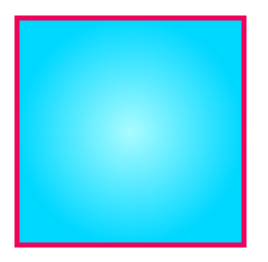2. Classify the following curves as open or closed: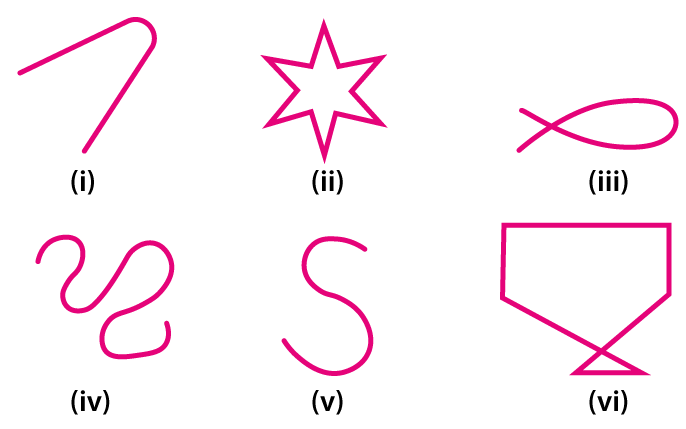Solution:

(i) From the figure we know that it is an open curve.

(ii) From the figure we know that it is a closed curve.

(iii) From the figure we know that it is a closed curve.

(iv) From the figure we know that it is an open curve.

(v) From the figure we know that it is an open curve.

(vi) From the figure we know that it is a closed curve.

3. Draw a polygon and shade its interior. Also draw its diagonals, if any.

Solution:

We know that ABCD is a polygon which contains two diagonals AC and BD.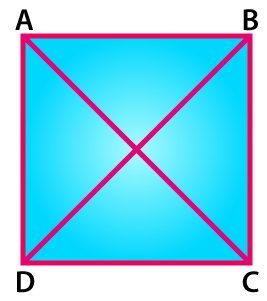4. Illustrate, if possible, each one of the following with a rough diagram:

(i) A closed curve that is not a polygon.

(ii) An open curve made up entirely of line segments.

(iii) A polygon with two sides.

Solution:

(i) A closed curve that is not a polygon is a circle which has only a curve.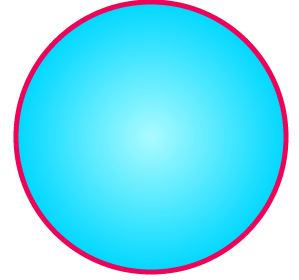(ii) An open curve made up entirely of line segments.(iii) A polygon with two sides is not possible.